+

# AVG to Average Values

##### Rating:
(0)
Author: Sophia Tutorial
##### Description:

Compose a query that shows the average of a given column within a table.

(more)
Tutorial

what's covered
This tutorial explores using the AVG function to average values of a given column within a table in two parts:
1. Introduction to the AVG Function
2. Dealing with 0 and NULL

## 1. Introduction to the AVG Function

The AVG function is used to calculate the average value of a set of values. This is one of the most commonly used aggregate functions. If we wanted to find the average total from the invoice table, we could run something like this:

``````
SELECT AVG(total)
FROM invoice;
``````

This would return a value like: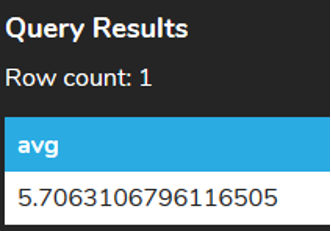This may not be entirely readable and being that the total is a price, it may make more sense to limit the result to two decimal places. Unique to PostgreSQL, we can cast the result as follows:

``````
SELECT AVG(total)::numeric(10,2)
FROM invoice;
``````

Above, we will be casting it to 10 digits with two digits after the decimal: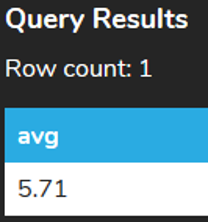In other databases, you may see the use of functions like ROUND to round the value or TRUNC to truncate the value to a certain position without rounding.

There are times that we may want to find averages of values with criteria being applied. For example, if we looked at all of the orders that were set by customer_id equal to 2:

``````
SELECT *
FROM invoice
WHERE customer_id = 2;
``````

We should find seven rows with a total value in each: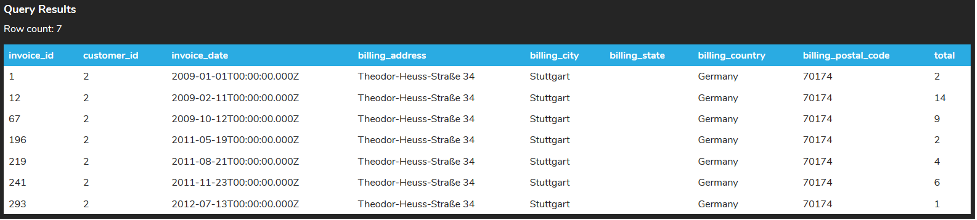If we calculated the average to two decimal places, it would look like this:

``````
SELECT AVG(total)::numeric(10,2)
FROM invoice
WHERE customer_id = 2;
``````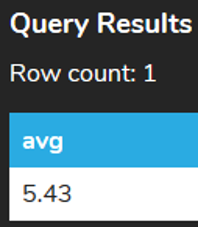## 2. Dealing with 0 and NULL

What is important to note is that the average only consists of values that are not NULL. For example, consider if we set one of the seven invoices to have the total as NULL:

``````
UPDATE invoice
SET total = NULL
WHERE invoice_id = 1;
``````

If we queried for the customer_id = 2, we would see that the total has no value. This is different than having a value of 0.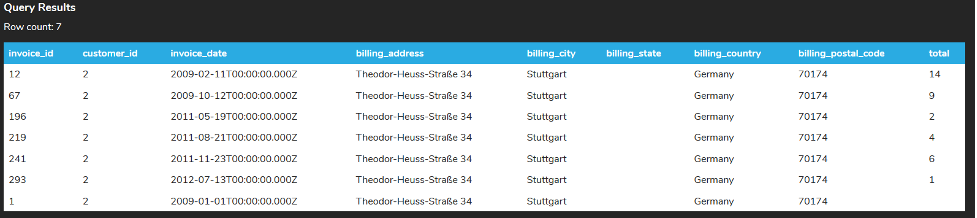Calculating the average would result in the following:

``````
SELECT AVG(total)::numeric(10,2)
FROM invoice
WHERE customer_id = 2;
``````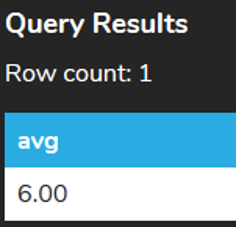However, if we updated that same invoice to set the total to 0 like this:

``````
UPDATE invoice
SET total = 0
WHERE invoice_id = 1;
``````

Running the same calculation:

``````
SELECT AVG(total)::numeric(10,2)
FROM invoice
WHERE customer_id = 2;
``````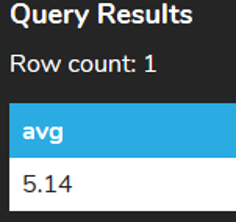In the first case, even though there are seven rows, the null value does not count so it does the following calculation (14 + 9 + 2 + 4 + 6 + 1)/6 = 6.00. In the second case, since the 0 is added instead of the null value, it counts it as part of the calculation as: (14 + 9 + 2 + 4 + 6 + 1 + 0)/7 = 5.14

Similar to the SUM function, if we only have NULL values, using the AVG would return NULL.

``````
SELECT AVG(total)
FROM invoice
WHERE invoice_id < 1;
``````For us to have a 0 returned, we would need to use the COALESCE function that would return the second argument if the first argument was NULL.

``````
SELECT COALESCE(AVG(total),0)
FROM invoice
WHERE invoice_id < 1;
``````try it
Your turn! Open the SQL tool by clicking on the LAUNCH DATABASE button below. Then enter in one of the examples above and see how it works. Next, try your own choices for which columns you want the query to provide.

summary
The AVG function allows us to calculate the average of a set of values.

Source: Authored by Vincent Tran

Rating Header